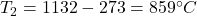## When doing numerical calculations involving temperature, you need to pay particular attention to the temperature scale you are using. In gen

Question

When doing numerical calculations involving temperature, you need to pay particular attention to the temperature scale you are using. In general, you should use the Kelvin scale (for which T = 0 represents absolute zero) in such calculations. This isbecause the standard thermodynamic equations (i.e., the ideal gaslaw and the formula for energy of a gas in terms of temperature)assume that zero degrees represents absolute zero.

If you are given temperatures measured in units other thankelvins, convert them to kelvins before plugging them into theseequations. (You may then want to convert back into the initialtemperature unit to give your answer.)
Part A) The average kinetic energy of the molecules of anideal gas at 10 ^\circ {\rm C} has the value K_10. At what temperature T_1 (in degrees Celsius) will the average kinetic energy ofthe same gas be twice this value, 2K_{10}?
Express the temperature to thenearest integer.
Part B) The molecules in an ideal gas at10 ^\circ {\rm C} have a root-mean-square (rms) speed v_rms. At what temperature T_2 (in degrees Celsius) will the molecules have twice therms speed, 2v_{\rm rms} ?

in progress 0
5 months 2021-08-14T23:58:46+00:00 1 Answers 8 views 0

1. 1)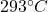2)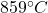Explanation:

1)

The average kinetic energy of the molecules of an ideal gas is directly related to the Kelvin temperature of the gas, by the formula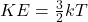where

KE is the kinetic energy

k is the Boltzmann constant

T is the Kelvin temperature

We can say  therefore that the average kinetic energy of the particles is directly proportional to the absolute temperature of the gas; so, we can write: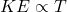And therefore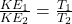(1)

In this problem, we have: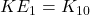is the initial kinetic energy of the molecules when the temperature of the gas is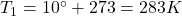Here we want to find the temperature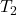at which the average kinetic energy of the particles is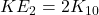So, twice the initial value. Substituting into eq.(1) and solving for T2, we find: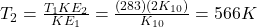Converting into Celsius degrees,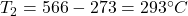2)

The root-mean-square (rms) speed of the molecules in a gas is given by the equation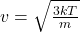where

k is the Boltzmann constant

T is the Kelvin temperature of the gas

m is the mass of each molecule

Therefore, from the equation we can say that the rms speed is proportional to the square root of the temperature: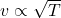So we can write: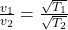(2)

where in this problem: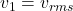is the rms speed of the molecules when the temperature is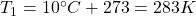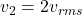is the final rms speed of the molecules

Solving eq.(2), we find the temperature at which the rms speed is twice the initial value: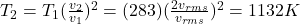Converting into Celsius degrees,